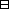## Group Theory and Linear Algebra

Last updated: 25 September 2014

## Lecture 31: Revision: Analogies

Let $G$ be a finite subgroup of $\text{Isom}\left({𝔼}^{2}\right)\text{.}$ Then $G$ is a cyclic or a dihedral group.Proof. Step 1 Let $p\in {𝔼}^{2}$ and $G=\left\{{g}_{1},\dots ,{g}_{t}\right\}\text{.}$ Then $q=g1p+⋯+grp$ is a fixed point of $G\text{.}$ So every element of $G$ is a rotation about $q$ or a reflection in a line through $q\text{.}$ Let $h={r}_{\theta ,q}$ with $\theta$ minimum possible and let $H=⟨h⟩\text{.}$ Then $H$ is a cyclic group. Case 1: If $H=G$ then $G$ is cyclic. Case 2: If $H\ne G$ let ${s}_{1},{s}_{2}\in G$ such that ${s}_{2},{s}_{1}\notin H$ and ${s}_{1}\ne {s}_{2}$ then ${s}_{1}{s}_{2}\in H$ so ${s}_{1}\in H{s}_{2}^{-1}=H{s}_{2},$ since ${s}_{2}^{2}=1\text{.}$ So $G=\left\{H,{s}_{1}H\right\}$ with ${s}_{1}^{2}=1\text{.}$ So $G$ is a dihedral group. $\square$

### Groups

A group is a set $G$ with a function $G×G ⟶ G (g1,g2) ⟼ g1g2$ such that

 (a) If ${g}_{1},{g}_{2},{g}_{3}\in G$ then $\left({g}_{1}{g}_{2}\right){g}_{3}={g}_{1}\left({g}_{2}{g}_{3}\right),$ (b) There exists $1\in G$ such that if $g\in G$ then $g·1=g$ and $1·g=g\text{.}$ (c) If $g\in G$ then there exists ${g}^{-1}\in G$ such that $g{g}^{-1}=1$ and ${g}^{-1}g=1\text{.}$

Homomorphisms are for comparing groups.

A homomorphism from $G$ to $H$ is a function $f:G\to H$ such that

 (a) If ${g}_{1},{g}_{2}\in G$ then $f\left({g}_{1}{g}_{2}\right)=f\left({g}_{1}\right)f\left({g}_{2}\right)\text{.}$

Let $f:G\to H$ be a homomorphism. The kernel of $f$ is $ker f={g∈G | f(g=1)}$ and the image of $f$ is $im f={f(g) | g∈G}.$

### Examples of groups

Cyclic groups, Dihedral groups, Symmetric groups. $Sn = { σ:{1,…,n}→ {1,…,n} | σ is a bijection } = graphs with n top vertices and n bottom vertices such that each top dot is connected to exactly one bottom dot and each bottom dot is connected to exactly one top dot$ with product given by composition ${\sigma }_{1}{\sigma }_{2}=\begin{array}{c} {\sigma }_{1} {\sigma }_{2} \end{array}$ $S3 = { , , , , , } , S2 = { , } , S1 = {}$ and  Note that $A = { , , , } is a subgroup of S4, A = ⟨⟩, the group generated by .$ Also $B = ⟨ , ⟩ = { , , , , , , , }$ is a subgroup of ${S}_{4}\text{.}$

## Notes and References

These are a typed copy of Lecture 31 from a series of handwritten lecture notes for the class Group Theory and Linear Algebra given on October 18, 2011.